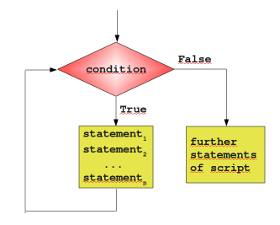# 循环

## Python 循环

Python中的循环语句有 for 和 while。

Python循环语句的控制结构图如下所示：## while 循环

Python中while语句的一般形式：

``````while 判断条件：
statements
``````

``````#!/usr/bin/env python3

n = 100

sum = 0
counter = 1
while counter <= n:
sum = sum + counter
counter += 1  print("Sum of 1 until %d: %d" % (n,sum))
``````

``````Sum of 1 until 100: 5050
``````

## for语句

Python for循环可以遍历任何序列的项目，如一个列表或者一个字符串。

for循环的一般格式如下：

``````for <variable> in <sequence>:
<statements>
else:
<statements>
``````

Python loop循环实例：

``````>>> languages = ["C", "C++", "Perl", "Python"]
>>> for x in languages:
...     print (x)
...
C
C++
Perl
Python
>>>
``````

``````#!/usr/bin/env python3
edibles = ["ham", "spam","eggs","nuts"]
for food in edibles:
if food == "spam":
break
print("Great, delicious " + food)
else:
print("I am so glad: No spam!")
print("Finally, I finished stuffing myself")
``````

``````Great, delicious ham
Finally, I finished stuffing myself
``````

## range()函数

``````>>> for i in range(5):
...     print(i)
...
0
1
2
3
4
``````

``````>>> for i in range(5,9) :
print(i)

5
6
7
8
>>>
``````

``````>>> for i in range(0, 10, 3) :
print(i)

0
3
6
9
>>>
``````

``````>>> for i in range(-10, -100, -30) :
print(i)

-10
-40
-70
>>>
``````

``````>>> a = ['Mary', 'had', 'a', 'little', 'lamb']
>>> for i in range(len(a)):
...     print(i, a[i])
...
0 Mary
2 a
3 little
4 lamb
``````

``````>>> list(range(5))
[0, 1, 2, 3, 4]
>>>
``````

## break和continue语句及循环中的else子句

break语句可以跳出for和while的循环体。如果你从for或while循环中终止，任何对应的循环else块将不执行。

continue语句被用来告诉Python跳过当前循环块中的剩余语句，然后继续进行下一轮循环。

``````>>> for n in range(2, 10):
...     for x in range(2, n):
...         if n % x == 0:
...             print(n, 'equals', x, '*', n//x)
...             break
...     else:
...         # 循环中没有找到元素
...         print(n, 'is a prime number')
...
2 is a prime number
3 is a prime number
4 equals 2 * 2
5 is a prime number
6 equals 2 * 3
7 is a prime number
8 equals 2 * 4
9 equals 3 * 3
``````

## pass语句

pass语句什么都不做。它只在语法上需要一条语句但程序不需要任何操作时使用.例如:

``````>>> while True:
...     pass  # 等待键盘中断 (Ctrl+C)
``````

``````>>> class MyEmptyClass:
...     pass
``````
JSRUN闪电教程系统是国内最先开创的教程维护系统， 所有工程师都可以参与共同维护的闪电教程，让知识的积累变得统一完整、自成体系。 大家可以一起参与进共编，让零散的知识点帮助更多的人。
X9.99